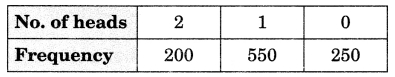# CBSEtips.in

## Saturday, 20 February 2021

### CBSE Class 9 Maths - MCQ and Online Tests - Unit 15 - Probability

#### CBSE Class 9 Maths – MCQ and Online Tests – Unit 15 – Probability

Every year CBSE  schools conducts Annual Assessment exams for 6,7,8,9,11th standards. These exams are very competitive to all the students. So our website provides online tests for all the 6,7,8,9,11th standard’s subjects. These tests are also very effective and useful for those who preparing for any competitive exams like Olympiad etc. It can boost their preparation level and confidence level by attempting these chapter wise online tests.

These online tests are based on latest CBSE syllabus. While attempting these our students can identify the weak lessons and continuously practice those lessons for attaining high marks. It also helps to revise the NCERT textbooks thoroughly.

#### CBSE Class 9 Maths – MCQ and Online Tests – Unit 15 – Probability

Question 1.
The sum of the probabilities of all events of a trial is
(a) 1
(b) greater than 1
(c) less than 1
(d) between 0 and 1

Question 2.
In a sample study of 640 people, it was found that 512 people have a high school certificate. If a person is selected at random, the probability that the person has a high school certificate is:
(a) 0.5
(b) 0.6
(c) 0.7
(d) 0.8

Question 3.
The probability that a number selected at random from the numbers 1, 2, 3, …, 15 is a multiple of 4 is
(a) $$\frac{4}{15}$$
(b) $$\frac{2}{15}$$
(c) $$\frac{1}{5}$$
(d) $$\frac{1}{3}$$

Answer: (c) $$\frac{1}{5}$$

Question 4.
In a survey of 364 children aged 19-36 months, it was found that 91 liked to eat potato chips. If a child is selected at random, the probability that he/she does not like to eat potato chips is:
(a) 0.25
(b) 0.50
(c) 0.75
(d) 0.80

Question 5.
Two coins are tossed 1000 times and the outcomes are recorded as below:The probability of getting at the most one head is:
(a) $$\frac{1}{5}$$
(b) $$\frac{1}{4}$$
(c) $$\frac{4}{5}$$
(d) $$\frac{3}{4}$$

Answer: (c) $$\frac{4}{5}$$

Question 6.
When a die is thrown, the probability of getting an odd number less than 4 is
(a) $$\frac{1}{6}$$
(b) $$\frac{1}{3}$$
(c) $$\frac{1}{2}$$
(d) 0

Answer: (b) $$\frac{1}{3}$$

Question 7.
A bag contains 16 cards bearing number 1, 2, 3 …., 16 respectively. One card is drawn at random. What is the probability that a number is divisible by 3?
(a) $$\frac{3}{16}$$
(b) $$\frac{5}{16}$$
(c) $$\frac{11}{16}$$
(d) $$\frac{13}{16}$$

Answer: (b) $$\frac{5}{16}$$

Question 8.
Which of the following cannot be the empirical probability of an event?
(a) $$\frac{2}{3}$$
(b) $$\frac{3}{2}$$
(c) 0
(d) 1

Answer: (b) $$\frac{3}{2}$$

Question 9.
A die is thrown 300 times and odd numbers are obtained 153 times. Then the probability of getting an even number is
(a) $$\frac{153}{300}$$
(b) $$\frac{147}{300}$$
(c) $$\frac{174}{300}$$
(d) $$\frac{147}{153}$$

Answer: (b) $$\frac{147}{300}$$

Share: